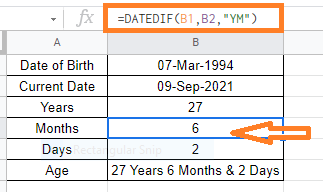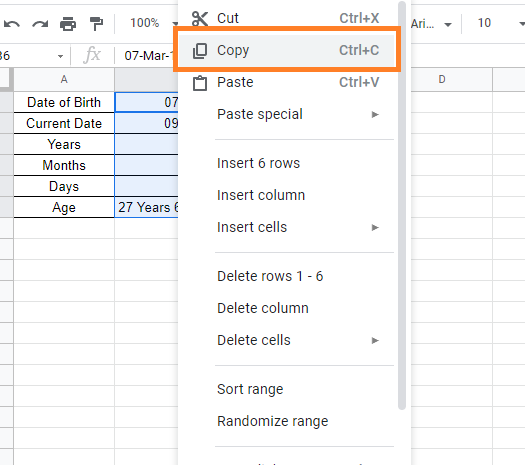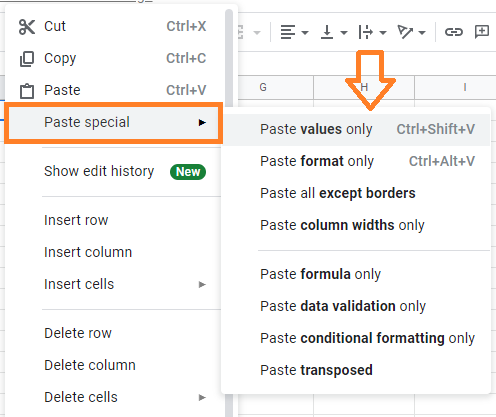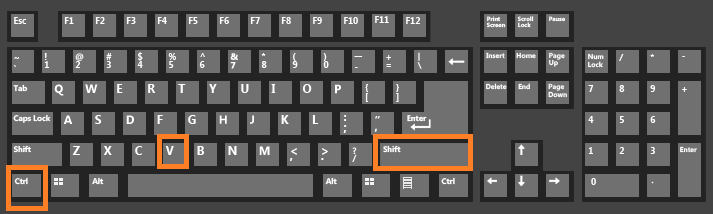## How to Convert Formula to Value in Google Sheets?

When working with Google Sheets, we use n number of formulas and functions. However, when we are copying formula applied cells from one cell to another cell, the Google Sheets will show up an error. This error will be shown due to the difference in cell reference numbers that we have used in formulas or functions. Thus in order to avoid this error, we should convert formulas to values in Google Sheets.

## How to Convert Formula to Value in Google Sheets?

In this post, let’s consider the following dataset as an example.

Now let’s understand how to convert formulas to values in Google Sheets with the help of the following two methods:

• Using Paste as Values
• Using Keyboard Shortcut

### Using Paste as Values to Convert Formulas to Values

Consider the following dataset where we need to convert formulas to values:Follow the steps listed below to convert formulas to values using the Paste as values method.

• Step 2: Now select the cells that is containing the formulas.
• Step 3: Use “CNTRL + C” to copy the selected cells. Alternatively, right-click and select “Copy“.• Step 4: Now move the cell, where you like to convert formulas to values.
• Step 5: Right-click and select “Paste Special“.
• Step 6: From the context sub-menu, select “Paste Values Only“.In this case, your formulas will be substituted with their returned values, and you will have no formulas at all.

### Using Keyboard Shortcut to Convert Formula to Value

Another easy method to convert formulas to values is using the keyboard shortcut.

Follow the steps listed below to convert formulas to values with the help of Keyboard shortcuts.

• Step 1: Select the cells whose formula needs to be converted to values.
• Step 2: Now copy the cells using “CNTRL+C”. If you are a mac user, press “CMD+C”.
• Step 3: CTRL+SHIFT+V is a keyboard shortcut that you can use (hold down the SHIFT and CTRL keys and then press the V key while the former two keys remain pressed). If you’re using a Mac, instead of CTRL, you can use the CMD key.### What is the Necessity to Convert Formulas to Values?

Converting a formula to a value can be useful in a variety of situations. The list of reasons why we need to convert formulas to values are explained below:

• When working with large spreadsheets, having too many formulas can cause the processing to slow down. Converting formulas to values can assist speed up spreadsheet processing if you don’t require them any longer.
• When you utilize functions like RAND or RANDBETWEEN, you’ll see that they automatically reload whenever the sheet changes. You might wish to limit yourself to one value. You may just convert the formula to value to prevent the randomly produced cell values from auto-refreshing every time.
• You might need to share a spreadsheet with someone but don’t want to reveal the formulas. It also aids in the conversion of formulas to values in such circumstances.